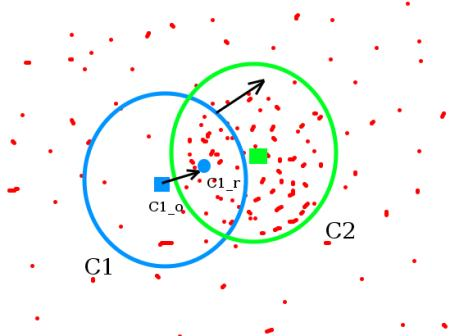# OpenCV-Python 系列 四十六 | Meanshift和Camshift

### 学习目标

– 我们将学习用于跟踪视频中对象的Meanshift和Camshift算法。

### Meanshift

Meanshift背后的直觉很简单，假设你有点的集合。（它可以是像素分布，例如直方图反投影）。你会得到一个小窗口（可能是一个圆形），并且必须将该窗口移到最大像素密度（或最大点数）的区域。如下图所示：### OpenCV中的Meanshift

import numpy as np
import cv2 as cv
import argparse
parser = argparse.ArgumentParser(description='This sample demonstrates the meanshift algorithm.
https://www.bogotobogo.com/python/OpenCV_Python/images/mean_shift_tracking/slow_traffic_small.mp4')
parser.add_argument('image', type=str, help='path to image file')
args = parser.parse_args()
cap = cv.VideoCapture(args.image)
# 视频的第一帧
# 设置窗口的初始位置
x, y, w, h = 300, 200, 100, 50 # simply hardcoded the values
track_window = (x, y, w, h)
# 设置初始ROI来追踪
roi = frame[y:y+h, x:x+w]
hsv_roi =  cv.cvtColor(roi, cv.COLOR_BGR2HSV)
mask = cv.inRange(hsv_roi, np.array((0., 60.,32.)), np.array((180.,255.,255.)))
cv.normalize(roi_hist,roi_hist,0,255,cv.NORM_MINMAX)
# 设置终止条件，可以是10次迭代，也可以至少移动1 pt
term_crit = ( cv.TERM_CRITERIA_EPS | cv.TERM_CRITERIA_COUNT, 10, 1 )
while(1):
if ret == True:
hsv = cv.cvtColor(frame, cv.COLOR_BGR2HSV)
dst = cv.calcBackProject([hsv],,roi_hist,[0,180],1)
# 应用meanshift来获取新位置
ret, track_window = cv.meanShift(dst, track_window, term_crit)
# 在图像上绘制
x,y,w,h = track_window
img2 = cv.rectangle(frame, (x,y), (x+w,y+h), 255,2)
cv.imshow('img2',img2)
k = cv.waitKey(30) & 0xff
if k == 27:
break
else:
break## Camshift### OpenCV中的Camshift

import numpy as np
import cv2 as cv
import argparse
parser = argparse.ArgumentParser(description='This sample demonstrates the camshift algorithm.
https://www.bogotobogo.com/python/OpenCV_Python/images/mean_shift_tracking/slow_traffic_small.mp4')
parser.add_argument('image', type=str, help='path to image file')
args = parser.parse_args()
cap = cv.VideoCapture(args.image)
# 获取视频第一帧
# 设置初始窗口
x, y, w, h = 300, 200, 100, 50 # simply hardcoded the values
track_window = (x, y, w, h)
# 设置追踪的ROI窗口
roi = frame[y:y+h, x:x+w]
hsv_roi =  cv.cvtColor(roi, cv.COLOR_BGR2HSV)
mask = cv.inRange(hsv_roi, np.array((0., 60.,32.)), np.array((180.,255.,255.)))
cv.normalize(roi_hist,roi_hist,0,255,cv.NORM_MINMAX)
# 设置终止条件，可以是10次迭代，有可以至少移动1个像素
term_crit = ( cv.TERM_CRITERIA_EPS | cv.TERM_CRITERIA_COUNT, 10, 1 )
while(1):
if ret == True:
hsv = cv.cvtColor(frame, cv.COLOR_BGR2HSV)
dst = cv.calcBackProject([hsv],,roi_hist,[0,180],1)
# 应用camshift 到新位置
ret, track_window = cv.CamShift(dst, track_window, term_crit)
# 在图像上画出来
pts = cv.boxPoints(ret)
pts = np.int0(pts)
img2 = cv.polylines(frame,[pts],True, 255,2)
cv.imshow('img2',img2)
k = cv.waitKey(30) & 0xff
if k == 27:
break
else:
break### 附加资源

1. French Wikipedia page on Camshift:http://fr.wikipedia.org/wiki/Camshift. (The two animations are taken from there)
2. Bradski, G.R., “Real time face and object tracking as a component of a perceptual user interface,” Applications of Computer Vision, 1998. WACV ’98. Proceedings., Fourth IEEE Workshop on , vol., no., pp.214,219, 19-21 Oct 1998

## Exercises

1. OpenCV comes with a Python :https://github.com/opencv/opencv/blob/master/samples/python/camshift.py for an interactive demo of camshift. Use it, hack it, understand it.